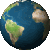# Is there a way to use a caret symbol in field calculator? ^?

371
3
10-13-2020 03:42 PMbyMVP

Here is the field calculator formula I'm trying to use. It's an Excel formula I've copied over and replaced referenced cells with columns in my table.

100-(15*(((0.445*((0.8*[SUM_t2])^1.15))+ (0.13 * ((0.8*[SUM_t1])^1.35)))^0.5))

1 Solution

Accepted SolutionsbyMVP Esteemed Contributor

^ in python is **, meaning to the power of, hence **0.5 is the sqrt

... sort of retired...
3 RepliesbyMVP Esteemed Contributor

^ in python is **, meaning to the power of, hence **0.5 is the sqrt

... sort of retired...byMVP

That did the trick. Thanks!byEsri Contributor

Not that I have ever come across, but I think what you could do is use either VBScript or Python and translate your Excel formula to python and use that in the field calculator to calculate the values.

Hope this helps.

Cheers,

Manish

If this answer solved your question or if you found it helpful please mark it accordingly to help others who have the same question.

Cheers,
Manish